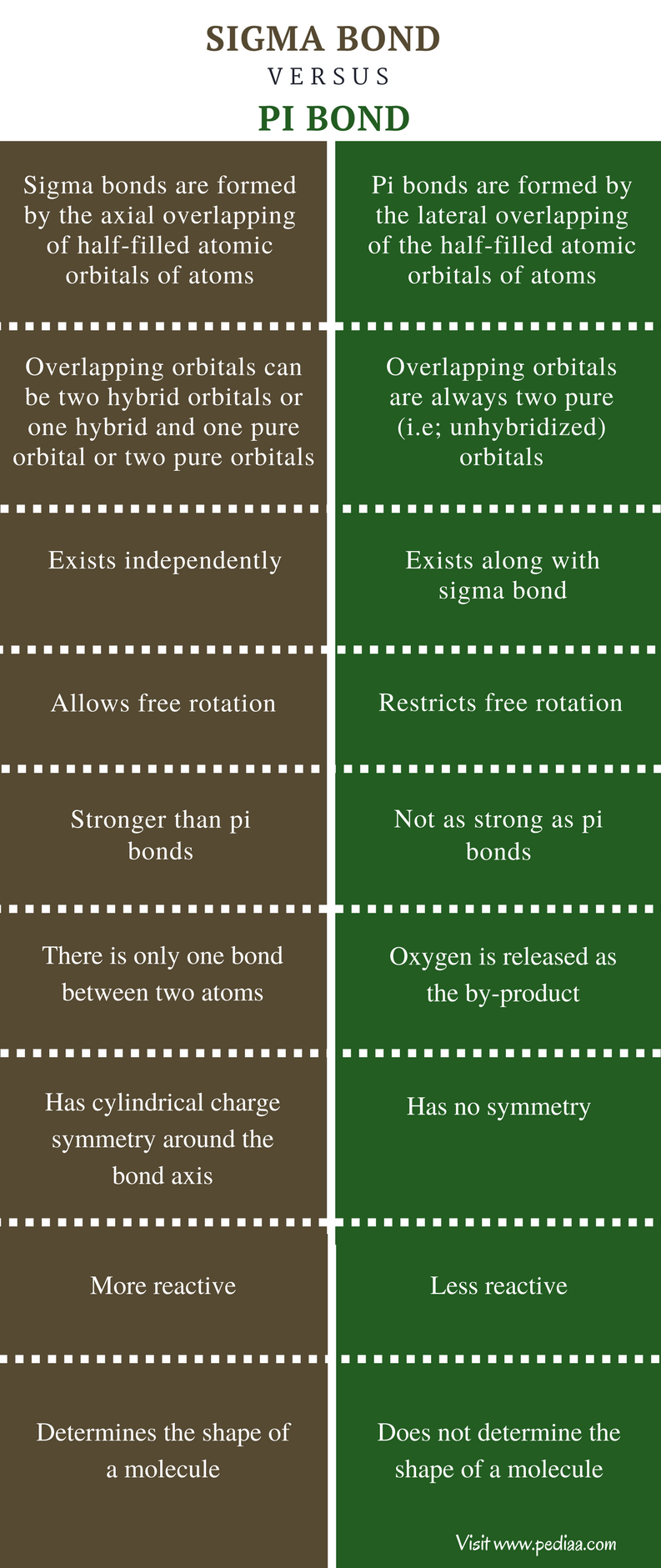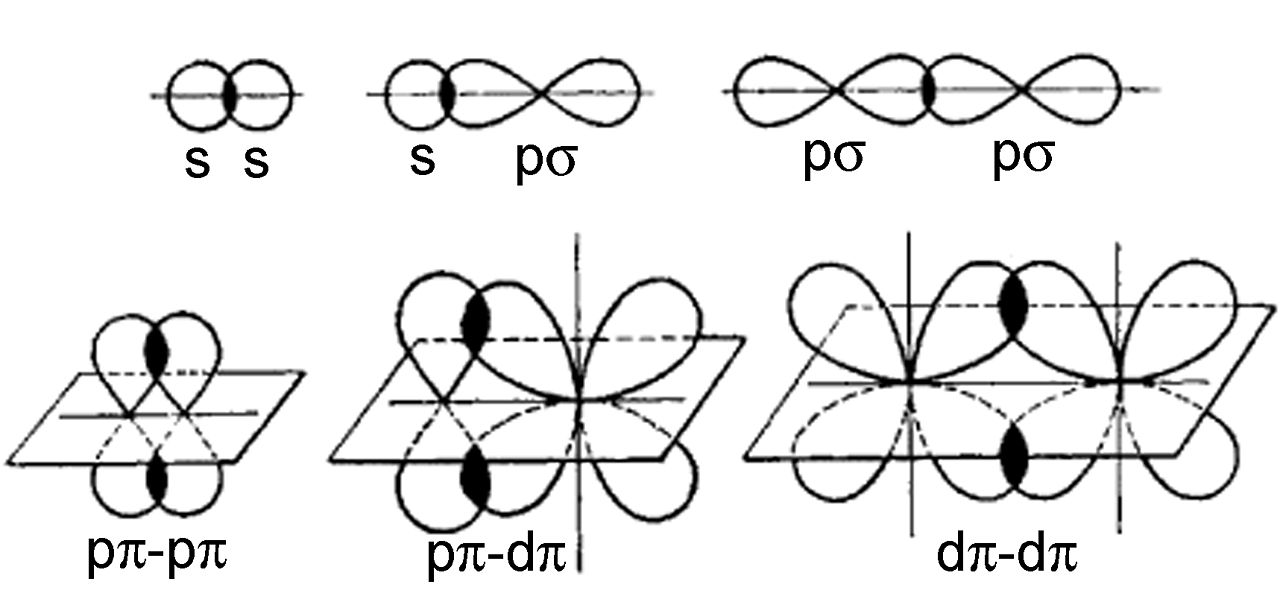# Difference Between Sigma and Pi Bond

## Main Difference – Sigma vs Pi Bond

Sigma and pi bonds are used to describe some features of covalent bonds and molecules with three or two atoms. These bonds are formed by overlapping of incomplete s and p orbitals of two atoms that participate for bonding. Hence, this model is often referred to as overlap model. The model is mainly applied to explain the bond formation of smaller atoms and is not applicable to explain the bonding of larger molecules. The main difference between sigma bond and pi bond is their formation; axial overlapping of two orbitals forms sigma bond while lateral overlapping of two orbitals forms pi bond.

1. What is a Sigma Bond
– Definition, Characteristics, Properties
2. What is a Pi Bond
– Definition, Characteristics, Properties
3. What is the difference between Sigma and Pi Bond## What is a Sigma Bond

The co-axial or linear overlapping of atomic orbitals of two atoms forms a sigma bond. It is the primary bond that is found in single, double and triple bonds. However, there can be only one sigma bond between two atoms. Sigma bond is stronger than pi bond because sigma bond has the maximum overlapping of atomic orbitals. It contains a single electron cloud, which lies along the bond axis. Sigma bond is the first bond to form during the formation of a covalent bond. Unlike pi bonds, both hybridized and unhybridized orbitals form sigma bonds.

## What is a Pi Bond

Pi bond is formed by lateral or sidewise or parallel overlapping of atomic orbitals. These bonds are weaker than sigma bonds because of the minimum extent of overlapping. Moreover, pi bonds are formed after the formation of sigma bonds. Hence, these bonds always exist with sigma bond. The pi bonds are formed by overlapping of unhybridized p-p atomic orbitals. Unlike sigma bonds, pi bonds do not affect the shape of a molecule. Single bonds are sigma bonds. But double and triple bonds have one and two pi bonds respectively, along with a sigma bond.Figure 01: Sigma bond and Pi bond

## Difference Between Sigma and Pi Bond

### Formation of Bonds

Sigma Bond: Sigma bonds are formed by the axial overlapping of half-filled atomic orbitals of atoms.

Pi Bond: Pi bonds are formed by the lateral overlapping of the half-filled atomic orbitals of atoms.

### Overlapping Orbitals

Sigma Bond: In sigma bonds, overlapping orbitals can be: two hybrid orbitals or one hybrid and one pure orbital or two pure orbitals

Pi Bond: In pi bonds, overlapping orbitals are always two pure (i.e; unhybridized) orbitals.

### Existence

Sigma Bond: Sigma bond exists independently.

Pi Bond: Pi-bond always exists along with sigma bond.

### Rotation of Two Carbon Atoms

Sigma Bond: Sigma bond allows free rotation.

Pi Bond: Pi bond restricts free rotation.

### Bond Strength

Sigma Bond: Sigma bonds are stronger than pi bonds.

Pi Bond: Pi bonds are less stronger than sigma bonds.

### Bond Forming Order

Sigma Bond: When atoms get closer, sigma bonds are formed first.

Pi Bond: Formation of pi bonds is preceded by the formation of sigma bonds.

### Number of Bonds

Sigma Bond: There is only one sigma bond between two atoms.

Pi Bond: There can be two pi bonds between two atoms.

### Control of Geometry in Polyatomic Molecules

Sigma Bond: Only sigma bonds are involved in the control of geometry in polyatomic molecules.

Pi Bond: Pi bonds are not involved in the control of geometry in polyatomic molecules.

### Number of bonds in a double bond

Sigma Bond: There is one sigma bond in a double bond.

Pi Bond: There is only one pi bond in a double bond.

### Number of bonds in a triple bond

Sigma Bond: There is one sigma bond in a triple bond.

Pi Bond: There are two pi bonds in a triple bond.

### Symmetry of the Charge

Sigma Bond: Sigma bond has cylindrical charge symmetry around the bond axis.

Pi Bond: Pi bond has no symmetry.

### Reactiveness

Sigma Bond: Sigma bonds are more reactive.

Pi Bond: Pi bonds are less reactive.

### Shape Determination

Sigma Bond: Shape of a molecule is determined by sigma bond.

Pi Bond:  Shape of a molecule is not determined by pi bond.

### Summary

Sigma and pi are two type of bonds formed due to the overlapping two atomic orbitals. Axial overlapping of two atoms forms a sigma bond, while lateral overlapping of two atomic orbitals forms a sigma bond. This is the key difference between sigma and pi bond. Sigma bond is always formed first and is stronger than pi bond. A single bond is always a sigma bond, while double bond and triple bond have one and two pi bonds respectively along with a sigma bond.

References
1. MOHAPATRA, R. K. (2014). ENGINEERING CHEMISTRY FOR DIPLOMA. PHI Learning Pvt. Ltd..
2. Srivastava, A. K. (2002). Organic Chemistry Made Simple. New Age International.
3. Jespersen, N. D., & Hyslop, A. (2014). Chemistry: The Molecular Nature of Matter: The Molecular Nature of Matter. Wiley Global Education.

Image Courtesy:
1. “Sigma and pi bonding” By Tem5psu – Own work (CC BY-SA 3.0) via Commons Wikimedia